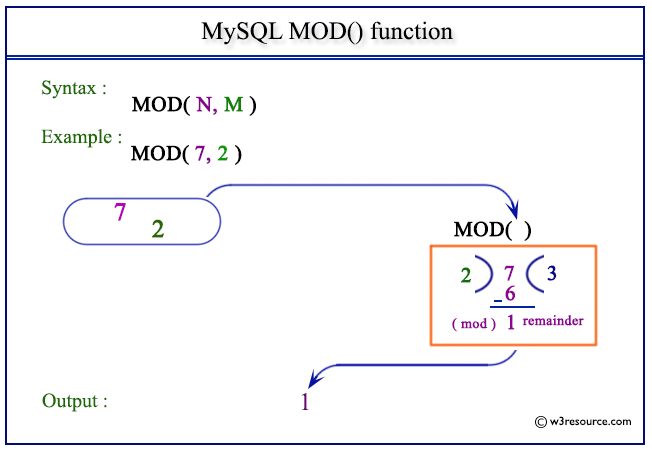# MySQL MOD() function

## MOD() function

MySQL MOD() returns the remainder of a number divided by another number. This function also works on fractional values and returns the exact remainder. The function returns NULL when the value of divisor is 0.

Syntax:

```MOD(N,M), N % M, N MOD M;
```

Arguments

Name Description
N Dividend.
M Divisor.

Pictorial presentation of MySQL MOD() functionExample of MySQL MOD() function

Code:

``````SELECT MOD(17,5);
```
```

Explanation

The above statement returns the remainder of 17 divided by 5.

Sample Output:

```mysql> SELECT MOD(17,5);
+-----------+
| MOD(17,5) |
+-----------+
|         2 |
+-----------+
1 row in set (0.00 sec)
```

Example : MOD() function using MOD keyword

Code:

``````SELECT 17 MOD 5;
```
```

Explanation

The above MySQL statement returns the remainder of 17 divided by 5. Notice that MOD keyword is used.

Sample Output:

```mysql> SELECT 17 MOD 5;
+----------+
| 17 MOD 5 |
+----------+
|        2 |
+----------+
1 row in set (0.00 sec)
```

Example : MOD() function using fractional value

Code:

``````SELECT MOD( 4.567, 2.42 );
```
```

Explanation

The above MySQL statement returns the remainder of the fractional number 4.567 divided by the fractional number 2.42.

Sample Output:

```mysql> SELECT MOD( 4.567, 2.42 );
+--------------------+
| MOD( 4.567, 2.42 ) |
+--------------------+
|              2.147 |
+--------------------+
1 row in set (0.00 sec)
```

Example : MOD() function using 0(zero) divisor

Code:

``````SELECT MOD( 13,0 );
```
```

Explanation

The above MySQL statement returns NULL because the value of the divisor is 0.

Sample Output:

```mysql> SELECT MOD( 13,0 );
+-------------+
| MOD( 13,0 ) |
+-------------+
|        NULL |
+-------------+
1 row in set (0.00 sec)
```

All Mathematical FunctionsPrevious: LOG10()
Next: OCT()

﻿